﻿ 具非线性来源项的抛物型方程反问题研究 An Parabolic Differential Equation Inverse Problem with a Nonlinear Source Term

Advances in Applied Mathematics
Vol.04 No.04(2015), Article ID:16300,6 pages
10.12677/AAM.2015.44040

An Parabolic Differential Equation Inverse Problem with a Nonlinear Source Term

Hui Sun, Cui’e Xiao

School of Mathematics and Computation Sciences, Hunan City University, Yiyang Hunan

Received: Oct. 18th, 2015; accepted: Nov. 2nd, 2015; published: Nov. 9th, 2015

Copyright © 2015 by authors and Hans Publishers Inc.

This work is licensed under the Creative Commons Attribution International License (CC BY).

http://creativecommons.org/licenses/by/4.0/ABSTRACT

This paper is devoted to a class of inverse problem for parabolic differential equation with a nonlinear source term. Using the theories of monotone operator and the Sobolev embedding theorem, we verify the existence and uniqueness of weak solution for the direct problem as well as the existence of quasi-solutions of the inverse source problem in an appropriate class of admissible source functions.

Keywords:Nonlinear Parabolic Differential Equation, Direct Problem, Existence, Uniqueness, Inverse Problem, Quasi-Solution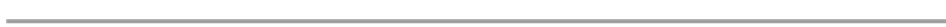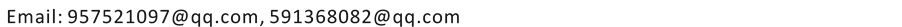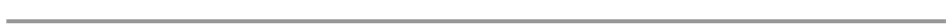1. 方程及基本假设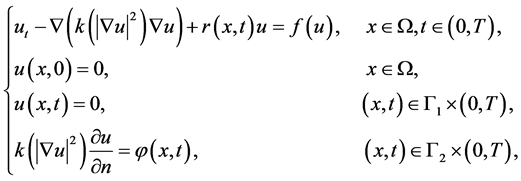(1.1)

(A1) 泛函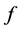有界，即把有界集映为有界集；

(A2) 函数满足Lipschitz条件，即存在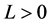，使得对任意的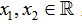，都有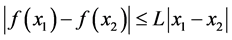.

(A3)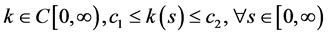(A4)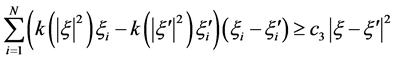(A5) 存在正常数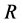，使得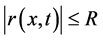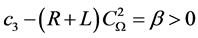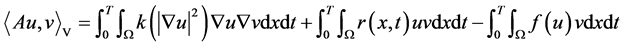。 (1.2)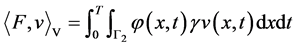。 (1.3)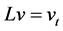。 (1.4)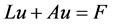。 (1.5)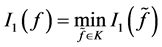。 (1.6)

2. 正问题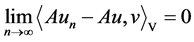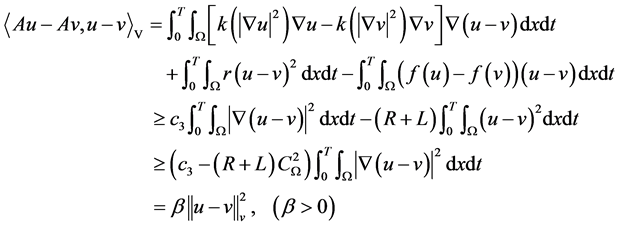(2.1)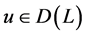,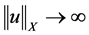时，。(2.2)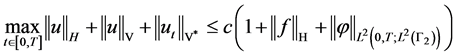(2.3)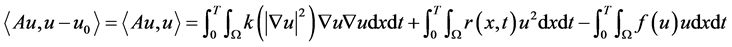(2.4)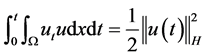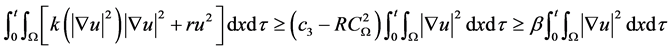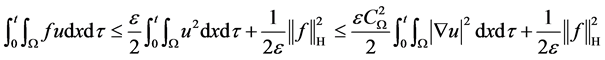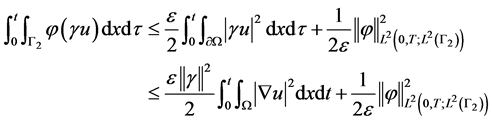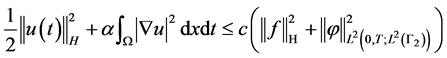(2.5)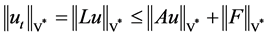， (2.6)

3. 反问题拟解的存在性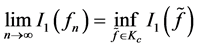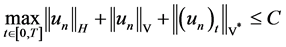。 (3.1)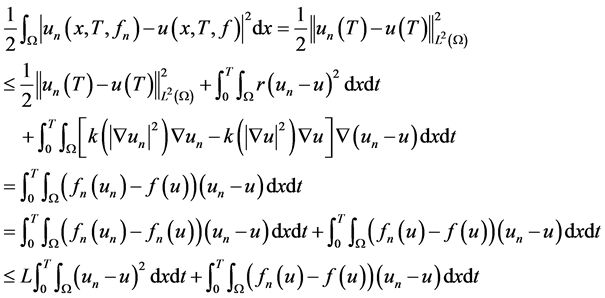(3.2)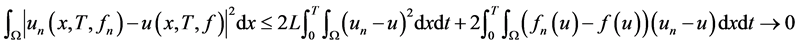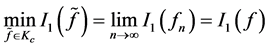An Parabolic Differential Equation Inverse Problem with a Nonlinear Source Term[J]. 应用数学进展, 2015, 04(04): 320-325. http://dx.doi.org/10.12677/AAM.2015.44040

1. 1. Xiao, C.E., Liu, J.B. and Liu, Y.L. (2011) An Inverse Pollution Problem in Porous Media. Applied Mathematics and Com-putation, 218, 3649-3653. http://dx.doi.org/10.1016/j.amc.2011.09.006

2. 2. 李景. 不适定问题的正则化方法[D]: [博士学位论文]. 长沙: 中南大学, 2009.

3. 3. Liu, Z.H. and Wang, B.Y. (2009) Coefficient Identification in Parabolic Equa-tions. Applied Mathematics and Computation, 209, 379-390. http://dx.doi.org/10.1016/j.amc.2008.12.062

4. 4. Hua, O.Y., Hasanov, A. and Hai, L.Z. (2008) Inverse Coefficient Problems for Nonlinear Parabolic Differential Equations. Acta Mathematica Sinica, English Series, 24, 1617-1624. http://dx.doi.org/10.1007/s10114-008-6384-0

5. 5. Liu, Z.H. (2000) On the Solvability of Degenerate Quasilinear Parabolic Equations of Second Order. Acta Mathematica Sinica, 16, 313-324. http://dx.doi.org/10.1007/s101140000052

6. 6. Liu, Z.H. (2005) On Doubly Degenerate Quasilinear Parabolic Equations of Higher Order. Acta Mathematica Sinica, 21, 197-208. http://dx.doi.org/10.1007/s10114-004-0415-2

7. 7. Liu, Z.H. (1999) Identification of Parameters in Semilinear Parabolic Equations. Acta Mathematica Scientia, 19, 175- 180.

8. 8. Zeidler, E. (1990) Nonlinear Functional Analysis and Its Applications II A/B. Springer, New York.

9. 9. Tikhonov, A. and Arsenin, V. (1977) Solutions of Ill-Posed Problems. Wiley, New York.